# How to use the Google Sheets SORT functionThe Google Sheets SORT function allows you to sort data and return the rows in a range either in ascending or descending order. You can use it to sort data alphabetically, numerically, or even by date, and you can sort both vertically and horizontally.

The SORT function also allows you to add multiple criteria across columns, in a similar way to the “Sort Range” functionality in the Google Sheets menu bar. The difference is that with the SORT function you can generate a new set of data while the original data remains intact.

SORT is also helpful when working with other functions such as LOOKUP that only work with sorted data.

### Syntax

=SORT(range, sort_column, is_ascending, [sort_column2, is_ascending2, …])

• range – is the reference to the group of cells that the SORT function uses.  It will sort the rows within the range as specified in the following two parameters:
• sort_column – is the column that the data will be sorted by. You can specify the column in two ways:
• An index number to specify a column number in the range which Google Sheets needs to sort (e.g. a sort_column value of 2 tells the function to sort the rows based on the values in the second column of the range).
• A reference (or address) of a column within the range of cells that you want to sort. Please note the column reference should include one single column that covers all the existing rows within the range. Otherwise, the function returns an #N/A! error.
• If you don’t input a value, the function assumes that the sort column is column 1 (i.e. the first column in the range).
• is_ascending – takes the values TRUE or FALSE to specify the order of data arrangement. It will sort the data in ascending order if TRUE, and in descending order if the option is FALSE. If you don’t input a value, the function automatically sorts the data in ascending order.
• sort_column2, is_ascending2, …
• These are optional parameters that can be used to do a multiple column sort. The syntax for these parameters remains the same as the ones above.

### How to use the Google Sheets SORT function

To demonstrate the benefits of the SORT function, I will be showing both the reference data as well as the resulting data that Google Sheets returns. Below are some of the main ways to use the SORT function.

#### Example 1: Basic sorting

Syntax: =SORT(range, column_index, ascending_order)

This is the most basic example of how to use the SORT function.

I have some data and I want to sort it alphabetically by name.

I do this by typing in =sort(A3:C16,2,True). As you can see, I am sorting the range A3:C16 on the second (Name) column in ascending order. The function rearranges the rows of data to give me the alphabetical order that I want.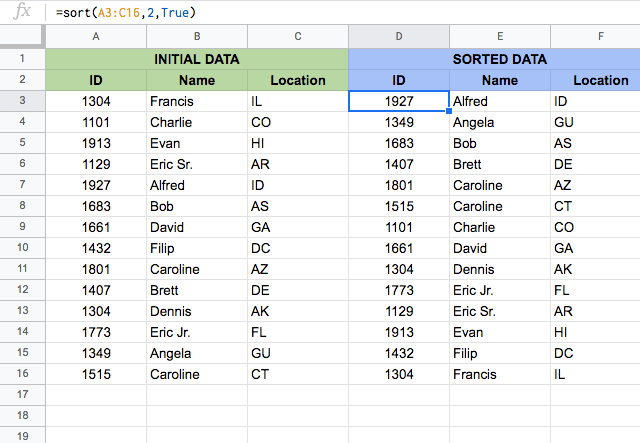I have typed in the function in cell D3, and the one thing to notice is that the function outputs the data from cell D3 onwards (down and to the right).

The number of columns and rows this function returns is the same as that of the input range. So it is very important to keep the cells where the data is expected to flow, clear of any values. Otherwise, the function returns a #REF! error, as you can see below.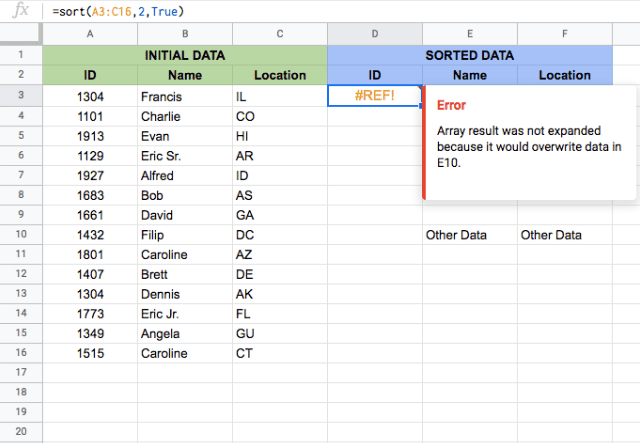#### Example 2: Multiple column sorting

Syntax: =SORT(range, column_index1, ascending_order1, column_index2, descending_order2)

Here I want to sort the data by multiple columns. When sorting by multiple columns, the first column that you specify (column_index1) takes priority, and the function sorts the data by the values in this column, just like in the previous example.

The function then looks at the second sort column and performs any sorting by that column that can be carried out without breaking the rules of the first column sorting.

In the example below, I first sort the range in ascending order on the second (Name) column, and then in ascending order on the first (ID) column. I do this by typing =sort(A3:C16,2,True,1,True).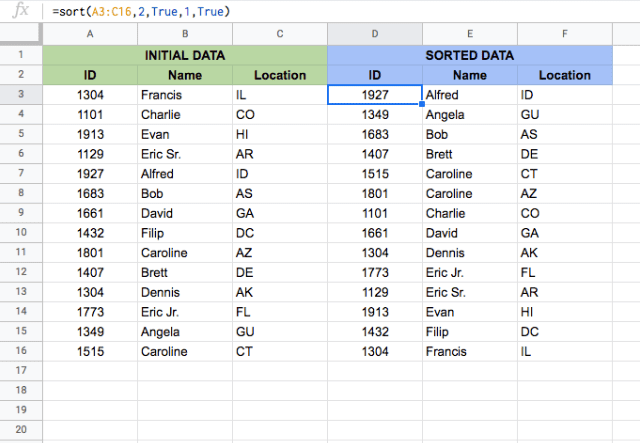Notice that the second sort (by ID) caused the two Carolines to change order. The Caroline with the numerically lower ID number was rearranged to be before the other Caroline.

This is the effect of a multiple sort: the first sort (by name) has priority, and the second sort (by ID number) only affects rows that can be rearranged while remaining properly sorted by name.

#### Example 3: Sorting with Column References

Syntax: =SORT(range, column_reference1, ascending_order1, column_reference2, descending_order2)

Up to this point, I’ve used the column index number to tell the function which column I want the data to be sorted by.

Now, for the sort_column parameter, I’ll try inputting the column references instead of column indexes. To do this, I type =sort(A3:C16,B3:B16,False,A3:A16,True).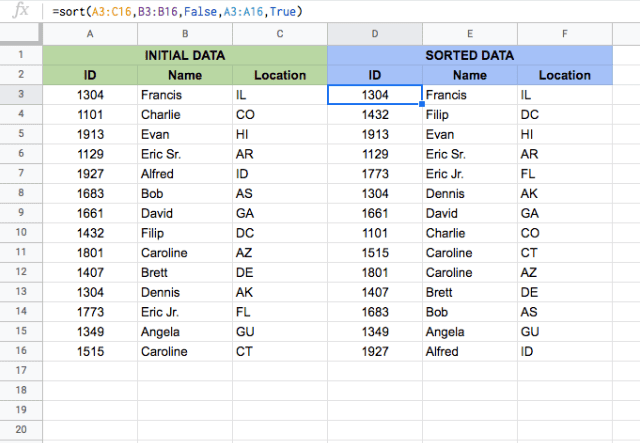Notice how the data is sorted by name in reverse alphabetical order – that’s because I typed FALSE for the ascending_order1 parameter, which tells the function to sort in descending order.

What happens when the column reference doesn’t cover all the rows within the range? Let’s try that (hint: SORT function returns an #N/A! error!). It also returns an error if the column reference is larger than the range.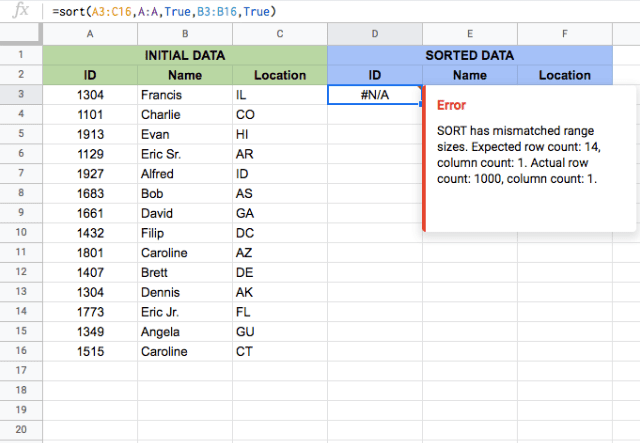#### Example 4: Sorting by date

Syntax: SORT(range, column_index, ascending_order)

Another convenient use of the SORT function is its ability to sort by date. Because the date in a spreadsheet is essentially just a number, you’re able to sort by date in exactly the same way you would sort numerically or alphabetically.

In the example below, I’ve added a column with some dates, and I’ll sort from oldest to most recent date by typing in =sort(A3:C16,3,True).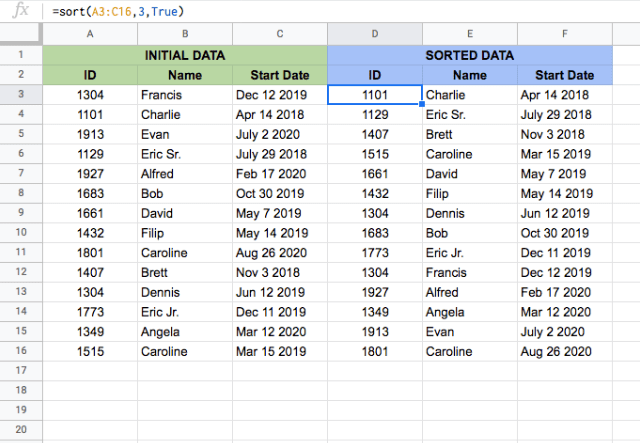#### Example 5: Horizontal sorting

Syntax: =Transpose(SORT(Transpose(range,) column_index, ascending_order))

While a vertically listed data set is common, there are of course times when you may have data that is listed horizontally.

Fortunately, the SORT function can still be used for horizontal data sets! All you have to do is use the TRANSPOSE function in combination with the SORT function.

The TRANSPOSE function transposes the rows and columns of an array, essentially flipping them. This is required because the SORT function is designed to use data in a vertical format. By transposing horizontally listed data, you get a list that the SORT function is able to work with.

Once it is sorted, it gets transposed back to its original horizontal format – that’s why the TRANSPOSE function is used twice!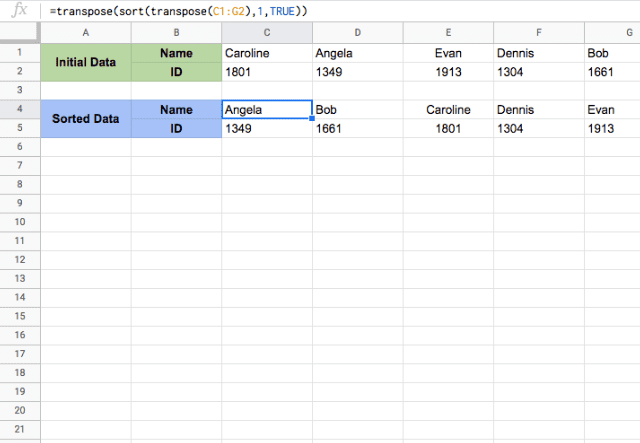In the above example, I’ve taken a horizontal data set and sorted it by typing =Transpose(SORT(Transpose(B1:F2),1,TRUE)).

#### Example 6: Sorting by a Range Outside the Sort Range

Syntax: SORT(range, column_reference, ascending_order)

There may be times when the value that you want to sort your data by is not actually included in the range of the data you’re sorting. This sounds complicated, but it is really quite a simple and convenient way that you can use the SORT function.

The example below will illustrate what I mean.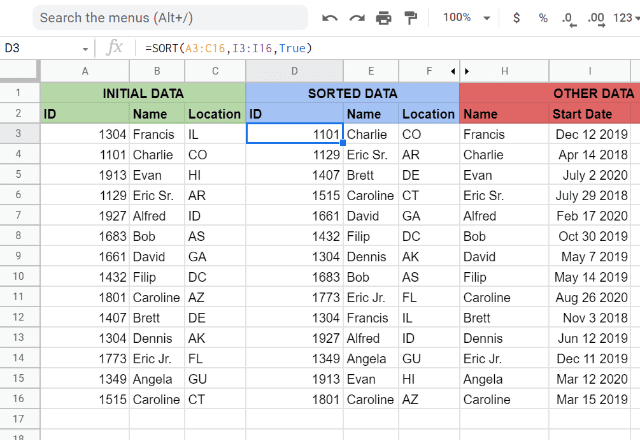In this example, there is some basic data, along with some additional data that shows the start date of each person.

To sort by start date, but without actually including the start date in the range (and therefore the output) of the SORT function, you simply reference the range of start dates as the column_index. I’ve done that here by typing =sort(A3:C16,I3:I16,True).

Notice that this function is the same as previous examples, with the only difference being that the sort range (in column I) is not actually within the data range (in columns A:C). Thankfully, the SORT function is still able to compute this, and it returns the list of names sorted by their start date.